# Seaborn入门：详解kdeplot和distplotSeaborn是基于matplotlib的Python可视化库。它提供了一个高级界面来绘制有吸引力的统计图形。Seaborn其实是在matplotlib的基础上进行了更高级的API封装，从而使得作图更加容易，不需要经过大量的调整就能使你的图变得精致。但应强调的是，应该把Seaborn视为matplotlib的补充，而不是替代物。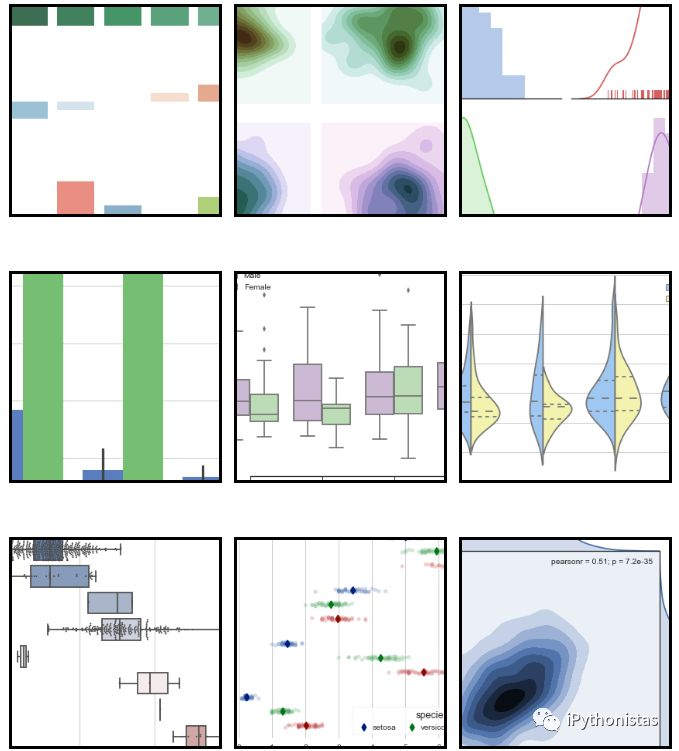Seaborn的安装

>>>pip install seaborn


#### kdeplot(核密度估计图)

%matplotlib inline  #IPython notebook中的魔法方法，这样每次运行后可以直接得到图像，不再需要使用plt.show()
import numpy as np  #导入numpy包，用于生成数组
import seaborn as sns  #习惯上简写成sns
sns.set()           #切换到seaborn的默认运行配置


x=np.random.randn(100)  #随机生成100个符合正态分布的数
sns.kdeplot(x)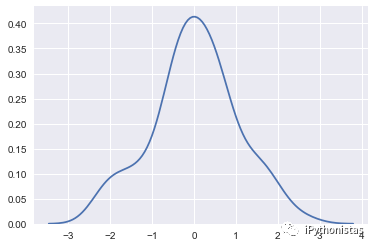cut：参数表示绘制的时候，切除带宽往数轴极限数值的多少(默认为3)

sns.kdeplot(x,cut=0)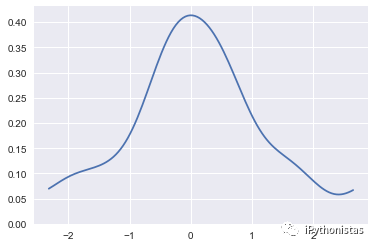cumulative ：是否绘制累积分布

sns.kdeplot(x,cumulative=True)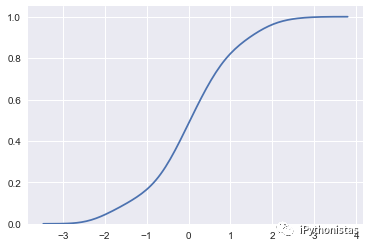sns.kdeplot(x,shade=True,color="g")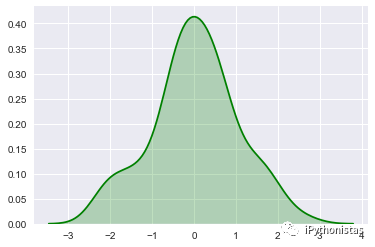vertical：表示以X轴进行绘制还是以Y轴进行绘制

sns.kdeplot(x,vertical=True)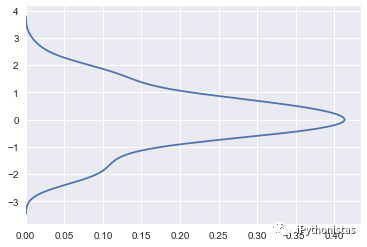y=np.random.randn(100)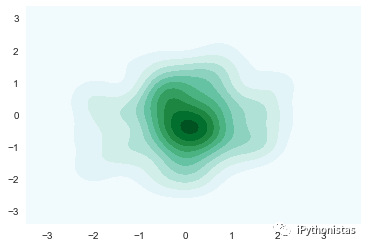cbar：参数若为True，则会添加一个颜色棒(颜色帮在二元kde图像中才有)

sns.kdeplot(x,y,shade=True,cbar=True)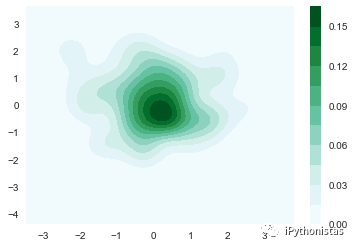#### distplot

displot()集合了matplotlib的hist()与核函数估计kdeplot的功能，增加了rugplot分布观测条显示与利用scipy库fit拟合参数分布的新颖用途。具体用法如下：

seaborn.distplot(a, bins=None, hist=True, kde=True, rug=False, fit=None, hist_kws=None, kde_kws=None, rug_kws=None, fit_kws=None, color=None, vertical=False, norm_hist=False, axlabel=None, label=None, ax=None)

sns.distplot(x,color="g")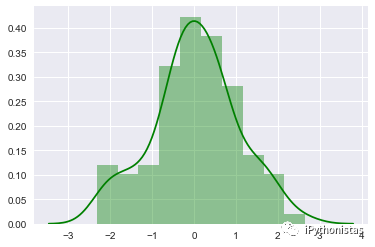import matplotlib.pyplot as plt
fig,axes=plt.subplots(1,3) #创建一个一行三列的画布
sns.distplot(x,ax=axes) #左图
sns.distplot(x,hist=False,ax=axes) #中图
sns.distplot(x,kde=False,ax=axes) #右图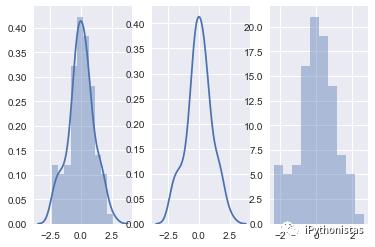bins：int或list，控制直方图的划分

fig,axes=plt.subplots(1,2)
sns.distplot(x,kde=False,bins=20,ax=axes) #左图：分成20个区间
sns.distplot(x,kde=False,bins=[x for x in range(4)],ax=axes) #右图：以0,1,2,3为分割点，形成区间[0,1],[1,2],[2,3]，区间外的值不计入。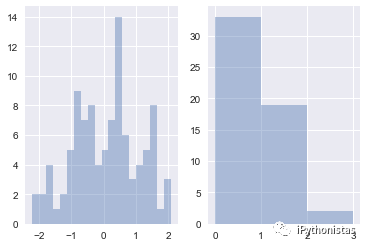rag：控制是否生成观测数值的小细条

fig,axes=plt.subplots(1,2)
sns.distplot(x,rug=True,ax=axes) #左图
sns.distplot(x,ax=axes) #右图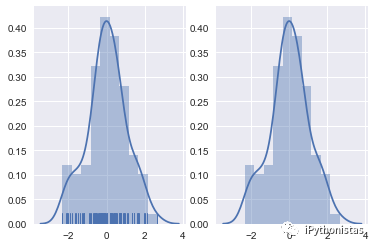fit：控制拟合的参数分布图形，能够直观地评估它与观察数据的对应关系(黑色线条为确定的分布)

from scipy.stats import *
sns.distplot(x,hist=False,fit=norm) #拟合标准正态分布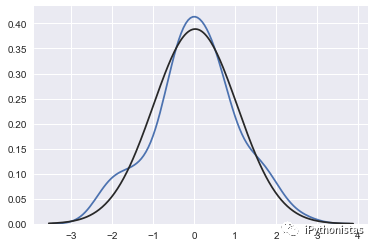hist_kws, kde_kws, rug_kws, fit_kws参数接收字典类型，可以自行定义更多高级的样式

sns.distplot(x,kde_kws={"label":"KDE"},vertical=True,color="y")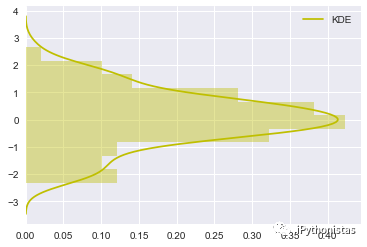norm_hist：若为True, 则直方图高度显示密度而非计数(含有kde图像中默认为True)

fig,axes=plt.subplots(1,2)
sns.distplot(x,norm_hist=True,kde=False,ax=axes) #左图
sns.distplot(x,kde=False,ax=axes) #右图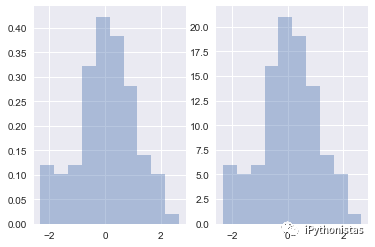▼点击成为社区注册会员      喜欢文章，点个

©️2019 CSDN 皮肤主题: 大白 设计师: CSDN官方博客